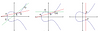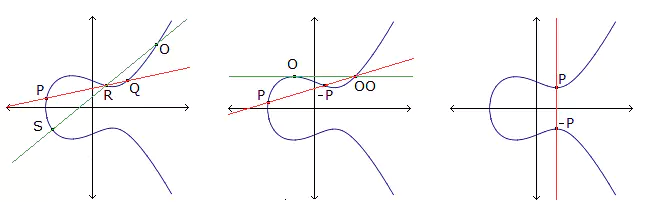# On cyclic caps in 4-dimensional projective spaces

### Journal article

M. Giulietti
Des. Codes Cryptogr., 2008

Semantic Scholar DBLP DOI
Cite

### Cite

APA
Giulietti, M. (2008). On cyclic caps in 4-dimensional projective spaces. Des. Codes Cryptogr.

Chicago/Turabian
Giulietti, M. “On Cyclic Caps in 4-Dimensional Projective Spaces.” Des. Codes Cryptogr. (2008).

MLA
Giulietti, M. “On Cyclic Caps in 4-Dimensional Projective Spaces.” Des. Codes Cryptogr., 2008.

## Abstract

For any divisor k of q4−1, the elements of a group of kth-roots of unity can be viewed as a cyclic point set Ck in PG(4,q). An interesting problem, connected to the theory of BCH codes, is to determine the spectrum A(q) of maximal divisors k of q4−1 for which Ck is a cap. Recently, Bierbrauer and Edel [Edel and Bierbrauer (2004) Finite Fields Appl 10:168–182] have proved that 3(q2 + 1)∈A(q) provided that q is an even non-square. In this paper, the odd order case is investigated. It is proved that the only integer m for which m(q2 + 1)∈A(q) is m = 2 for q ≡ 3 (mod 4), m = 1 for q ≡ 1 (mod 4). It is also shown that when q ≡ 3 (mod 4), the cap $$C_{2(q^{2}+1)}$$ is complete.

Share